# Chapter 4 Vector Spaces 4 1 Vector spaces

• Slides: 20Chapter 4 Vector Spaces • 4. 1 Vector spaces and Subspaces 向量空间 和 子空间 • 4. 2 Null Space and Column Space 零空间 和 列空间 • 4. 3 Linearly independent sets, bases • 4. 4 Coordinate systems 坐标系 • 4. 5 The dimension of a vector space 维数 • 4. 6 Rank 秩 1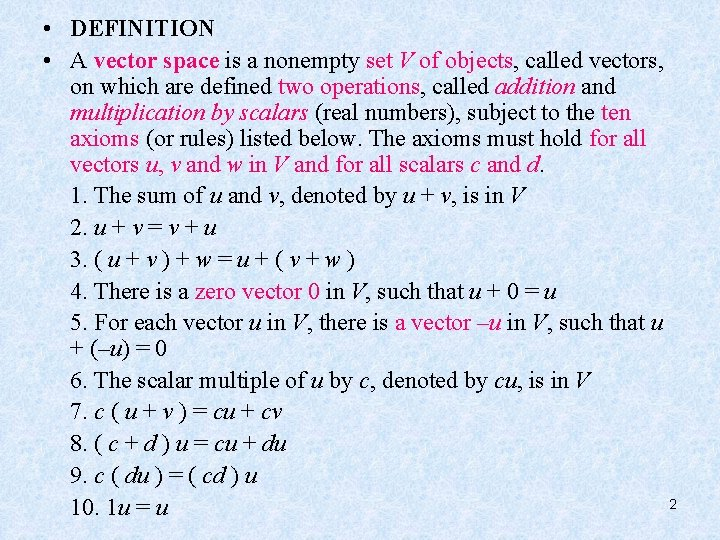• DEFINITION • A vector space is a nonempty set V of objects, called vectors, on which are defined two operations, called addition and multiplication by scalars (real numbers), subject to the ten axioms (or rules) listed below. The axioms must hold for all vectors u, v and w in V and for all scalars c and d. 1. The sum of u and v, denoted by u + v, is in V 2. u + v = v + u 3. ( u + v ) + w = u + ( v + w ) 4. There is a zero vector 0 in V, such that u + 0 = u 5. For each vector u in V, there is a vector –u in V, such that u + (–u) = 0 6. The scalar multiple of u by c, denoted by cu, is in V 7. c ( u + v ) = cu + cv 8. ( c + d ) u = cu + du 9. c ( du ) = ( cd ) u 2 10. 1 u = u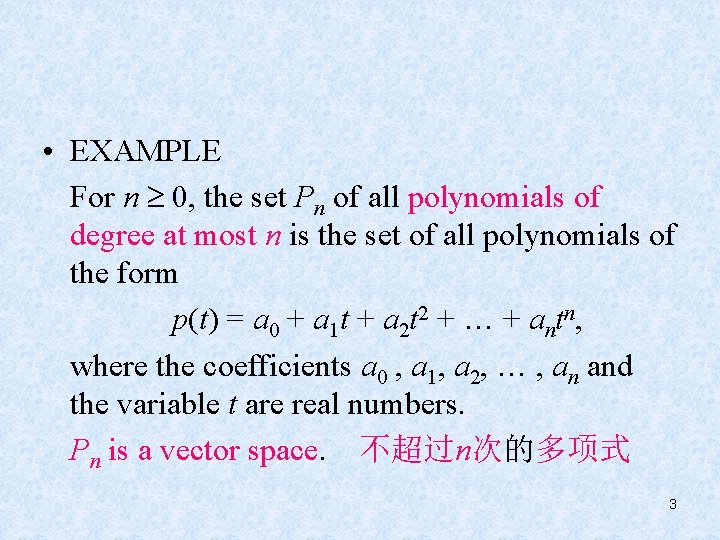• EXAMPLE For n 0, the set Pn of all polynomials of degree at most n is the set of all polynomials of the form p(t) = a 0 + a 1 t + a 2 t 2 + … + antn, where the coefficients a 0 , a 1, a 2, … , an and the variable t are real numbers. Pn is a vector space. 不超过n次的多项式 3• THEOREM 1 • If v 1, v 2 , …, vp are in a vector space V, then Span{v 1, v 2 , …, vp } is a subspace of V. 44. 2 Null Space and Column Space • The Null Space of a Matrix Nul A = {x : x Rn and Ax = 0 }. • If A=[a 1, …, an], then Col A = Span{ a 1, …, an } 5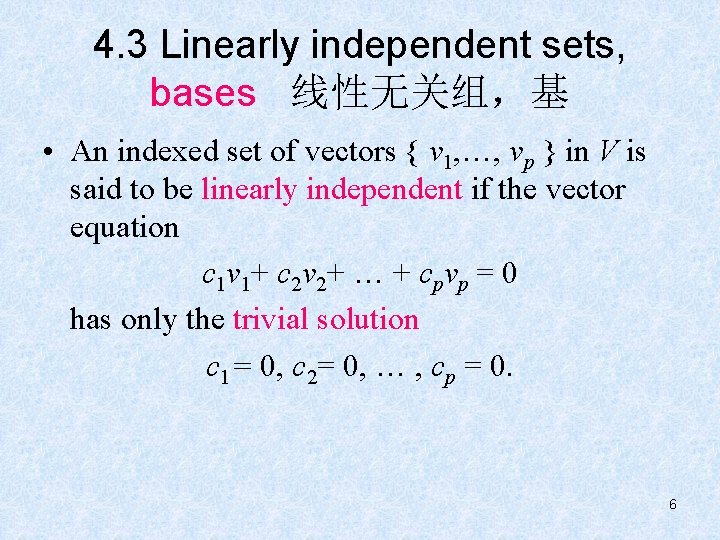4. 3 Linearly independent sets, bases 线性无关组，基 • An indexed set of vectors { v 1, …, vp } in V is said to be linearly independent if the vector equation c 1 v 1+ c 2 v 2+ … + cpvp = 0 has only the trivial solution c 1= 0, c 2= 0, … , cp = 0. 6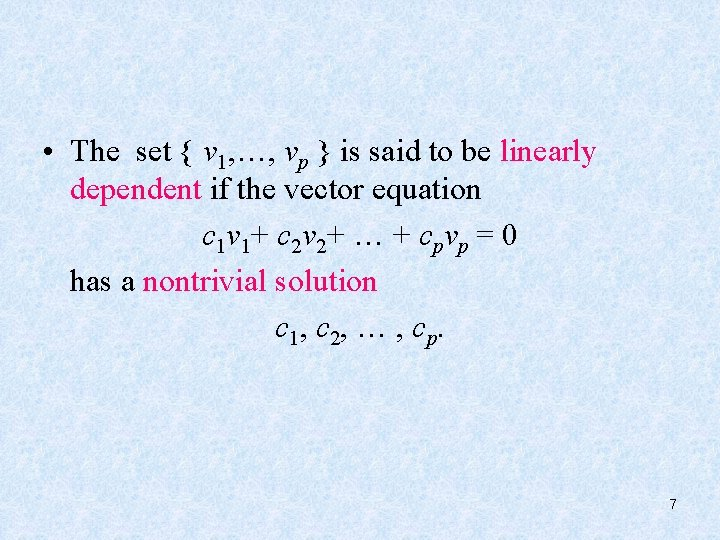• The set { v 1, …, vp } is said to be linearly dependent if the vector equation c 1 v 1+ c 2 v 2+ … + cpvp = 0 has a nontrivial solution c 1, c 2, … , cp. 7• THEOREM 4 • An indexed set {v 1, …, vp } of two or more vectors, with v 1 0, is linearly dependent if and only if some vj (with j >1) is a linear combination of the preceding vectors v 1, …, vj 1. • Proof c 1 v 1+ c 2 v 2+ … + cpvp = 0, …… 8• EXAMPLE 1 • Let p 1(t)=1, p 2(t)=t, and p 3(t)=4 -t. Then { p 1, p 2, p 3} is linearly dependent because p 3=4 p 1 -p 2. 9• DEFINITION • Let H be a subspace of a vector space V. An indexed set of vectors B={ b 1, …, bp } in V is a basis for H if (i) B is a linearly independent set, and (ii) the subspace spanned by B coincides with H, that is, H = Span{ b 1, …, bp }. 10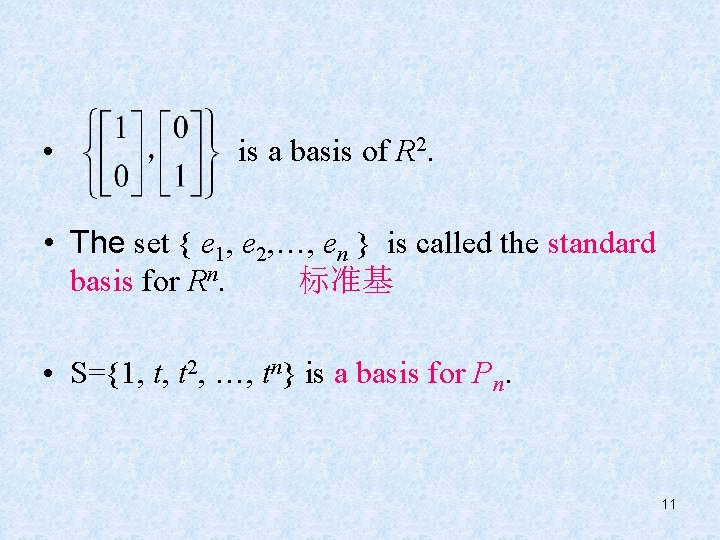• is a basis of R 2. • The set { e 1, e 2, …, en } is called the standard basis for Rn. 标准基 • S={1, t, t 2, …, tn} is a basis for Pn. 11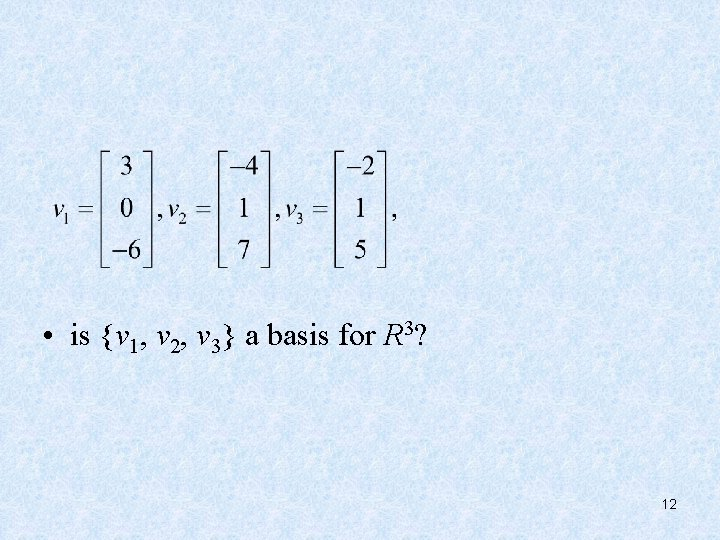• is {v 1, v 2, v 3} a basis for R 3? 12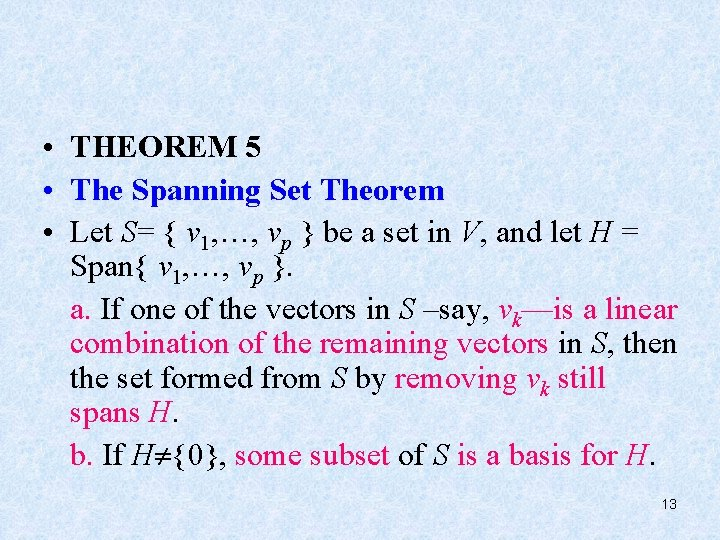• THEOREM 5 • The Spanning Set Theorem • Let S= { v 1, …, vp } be a set in V, and let H = Span{ v 1, …, vp }. a. If one of the vectors in S –say, vk—is a linear combination of the remaining vectors in S, then the set formed from S by removing vk still spans H. b. If H {0}, some subset of S is a basis for H. 13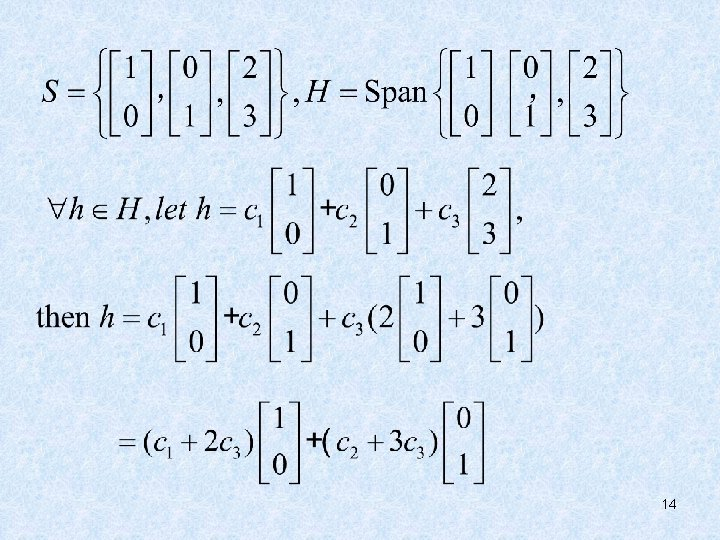14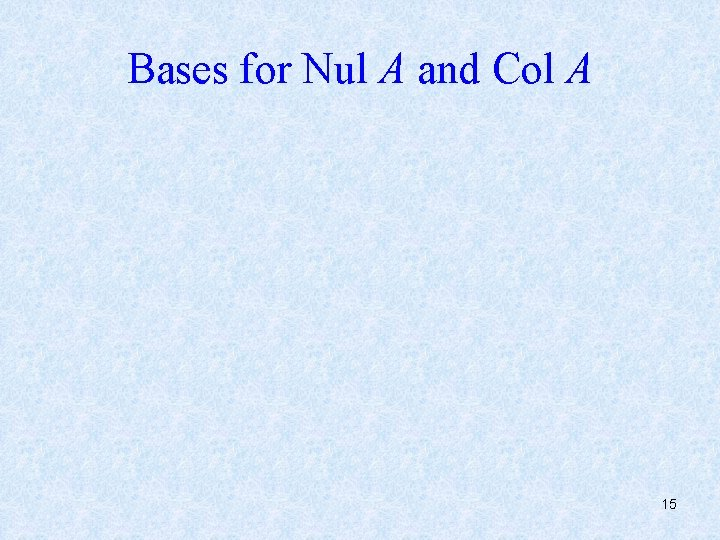Bases for Nul A and Col A 15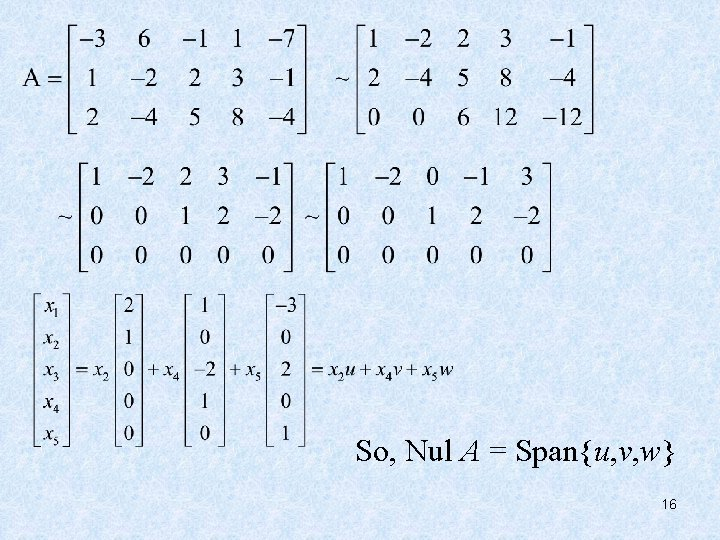So, Nul A = Span{u, v, w} 16is a basis of Nul A. 17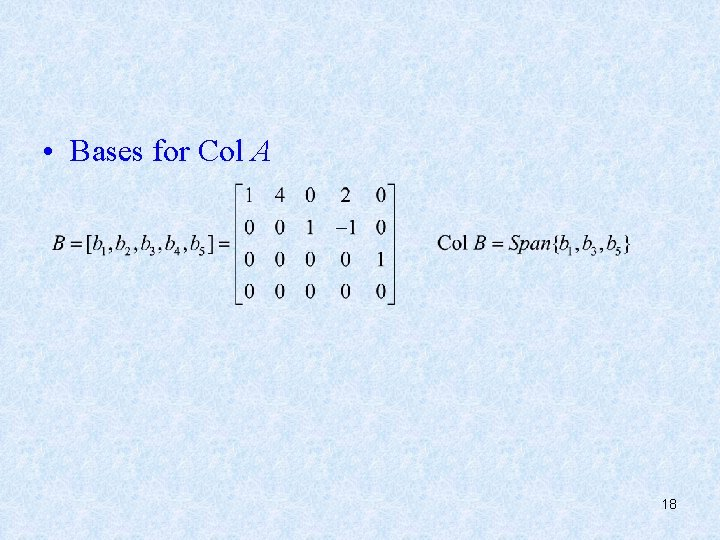• Bases for Col A 18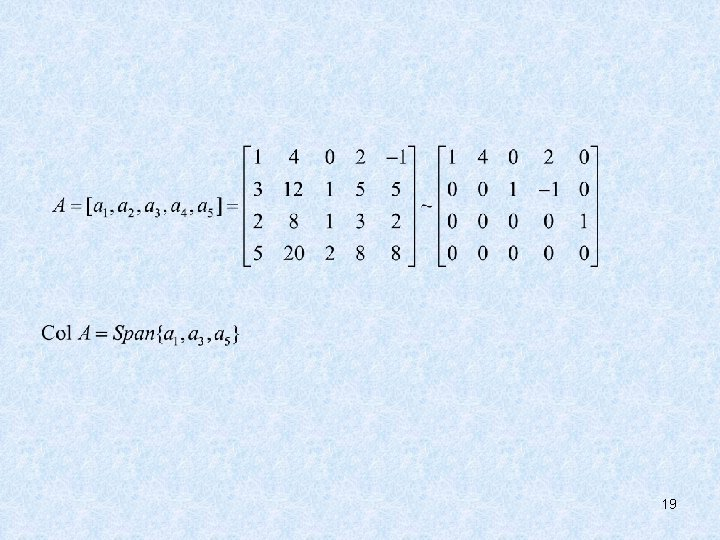19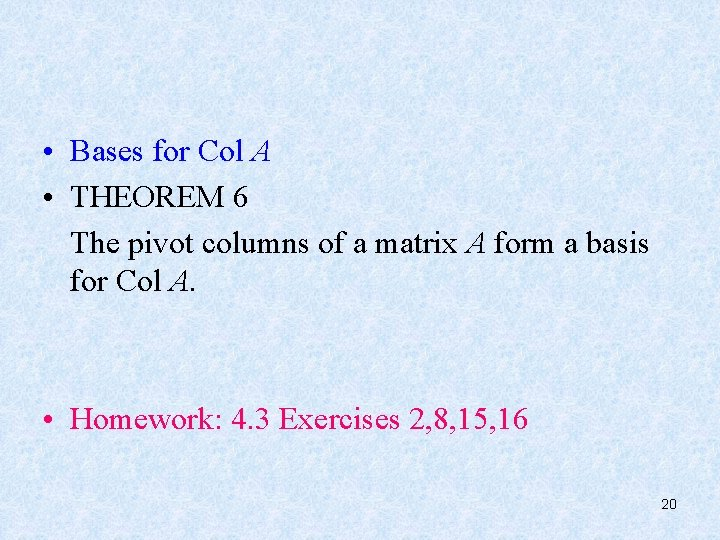• Bases for Col A • THEOREM 6 The pivot columns of a matrix A form a basis for Col A. • Homework: 4. 3 Exercises 2, 8, 15, 16 20